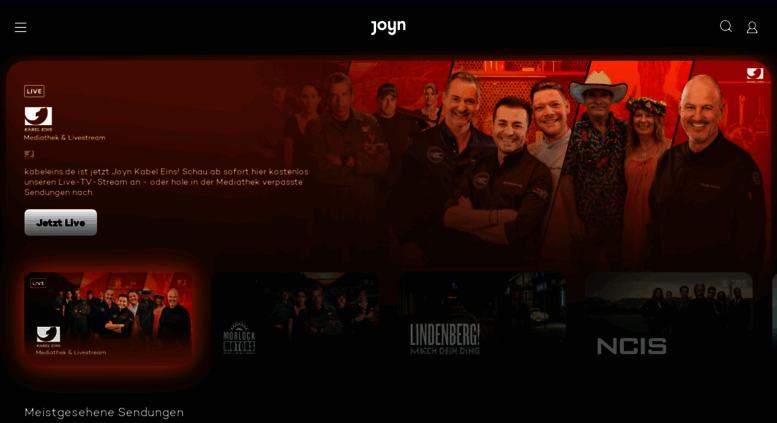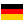# kabeleins.de: Kabel Eins - TV Programm, Sendungen, Livestream & Games

TV-Programm und beste Unterhaltung: Kabel Eins steht online für die besten Serien und Filme aller Zeiten, Livestream, Videos, Rezepte und kostenlose Games.

• 56/100

Normal result
• 6

Successful tests
• 2

Failed tests
• First response

208 ms

5.7 sec

• Page rendered

607 ms

6.5 sec

Click here to check amazing Kabel Eins content for Germany. Otherwise, check out these important facts you probably never knew about kabeleins.de

We analyzed Kabeleins.de page load time and found that the first response time was 208 ms and then it took 6.3 sec to load all DOM resources and completely render a web page. This is a poor result, as 80% of websites can load faster.

## Page optimization

•  HTML 238.5 kB Images 905.0 kB Javascripts 2.1 MB CSS 668.8 kB

In fact, the total size of Kabeleins.de main page is 3.9 MB. This result falls beyond the top 1M of websites and identifies a large and not optimized web page that may take ages to load. 75% of websites need less resources to load and that’s why Accessify’s recommendations for optimization and resource minification can be helpful for this project. Javascripts take 2.1 MB which makes up the majority of the site volume.

• Original 238.5 kB
• After minification 185.0 kB
• After compression 35.0 kB

HTML optimization

HTML content can be minified and compressed by a website’s server. The most efficient way is to compress content using GZIP which reduces data amount travelling through the network between server and browser. This page needs HTML code to be minified as it can gain 53.6 kB, which is 22% of the original size. It is highly recommended that content of this web page should be compressed using GZIP, as it can save up to 203.6 kB or 85% of the original size.

• Original 905.0 kB
• After optimization 886.3 kB

Image optimization

Image size optimization can help to speed up a website loading time. The chart above shows the difference between the size before and after optimization. Kabel Eins images are well optimized though.

• Original 2.1 MB
• After minification 2.1 MB
• After compression 637.3 kB

JavaScript optimization

It’s better to minify JavaScript in order to improve website performance. The diagram shows the current total size of all JavaScript files against the prospective JavaScript size after its minification and compression. It is highly recommended that all JavaScript files should be compressed and minified as it can save up to 1.5 MB or 70% of the original size.

• Original 668.8 kB
• After minification 664.4 kB
• After compression 119.2 kB

CSS optimization

CSS files minification is very important to reduce a web page rendering time. The faster CSS files can load, the earlier a page can be rendered. Kabeleins.de needs all CSS files to be minified and compressed as it can save up to 549.7 kB or 82% of the original size.

### Network requests diagram

• www.kabeleins.de
• k1.css
• stingray.js
• vendor.bundle.min.js
• ezone.min.js
• static.js
• coral.js
• gpt.js
• container.html
• utag.js
• bg_overlay.jpg
• bg.jpg
• analytics.js
• webtrekk.js
• iam.js
• ggcmb390.js
• kabel1de.js
• globalV6.js
• webtrekk-media.js
• rc
• c.ount
• gw.js
• pql
• pixel
• pixel
• default.js
• oba_icon.png
• 20417,82940,20409,157472,20413,24813
• 2b7eda3ec948b6c63fa7cb7891d9274
• p.min.js
• data
• c.ount
• www.kabeleins.de;fv=0;sx=1680;sy=1050;zz=1x1;site=DE_KABEL1;zone=home;idf=kabel1;c1=0;c2=0;c3=0;c4=1;c5=1;c6=0;c7=0;c8=0;c9=0;c10=0;c11=1;c12=0;c13=0;c14=0;c16=0;c17=0;c19=0;c20=0;v1=5;d1=1;d3=1;d4=0;d8=1;d9=3;d10=3;d12=3;d14=1;d15=5;d16=1;d17=1;d20=0;d21=1;d22=-1;i55=0;i11=1;i13=1;i21=1;i42=1;i44=1;i52=1;i53=1;n3=1;n5=1;n8=1;n9=0;f1=1;f2=1;f3=1;xx=pl;xx=fa;xx=pb;xx=pd;xx=bb;xx=wp;xx=fp;xx=sb;xx=pu1;xx=fb2;xx=rt1;xx=sc1;tile=1;ord=1347807380
• 172528364.js
• rs.js
• rpc.flow
• vce_st.js
• p2
• c.ount
• www.kabeleins.de;fv=0;sx=1680;sy=1050;zz=468x60;site=DE_KABEL1;zone=home;idf=kabel1;c1=0;c2=0;c3=0;c4=1;c5=1;c6=0;c7=0;c8=0;c9=0;c10=0;c11=1;c12=0;c13=0;c14=0;c16=0;c17=0;c19=0;c20=0;v1=5;d1=1;d3=1;d4=0;d8=1;d9=3;d10=3;d12=3;d14=1;d15=5;d16=1;d17=1;d20=0;d21=1;d22=-1;i55=0;i11=1;i13=1;i21=1;i42=1;i44=1;i52=1;i53=1;n3=1;n5=1;n8=1;n9=0;f1=1;f2=1;f3=1;xx=pl;xx=fa;xx=pb;xx=pd;xx=bb;xx=wp;xx=fp;xx=sb;xx=pu1;xx=fb2;xx=rt1;xx=sc1;tile=2;ord=1347807380
• p
• standard.js
• som-script.php
• 2.gif
• p
• rpc.flow
• p
• c.ount
• www.kabeleins.de;fv=0;sx=1680;sy=1050;zz=160x600;site=DE_KABEL1;zone=home;idf=kabel1;c1=0;c2=0;c3=0;c4=1;c5=1;c6=0;c7=0;c8=0;c9=0;c10=0;c11=1;c12=0;c13=0;c14=0;c16=0;c17=0;c19=0;c20=0;v1=5;d1=1;d3=1;d4=0;d8=1;d9=3;d10=3;d12=3;d14=1;d15=5;d16=1;d17=1;d20=0;d21=1;d22=-1;i55=0;i11=1;i13=1;i21=1;i42=1;i44=1;i52=1;i53=1;n3=1;n5=1;n8=1;n9=0;f1=1;f2=1;f3=1;xx=pl;xx=fa;xx=pb;xx=pd;xx=bb;xx=wp;xx=fp;xx=sb;xx=pu1;xx=fb2;xx=rt1;xx=sc1;tile=3;ord=1347807380
• p
• rpc.flow
• p
• NexaSlab-HeavyItalic.woff
• Nexa-HeavyItalic.woff
• t.js
• ph_k1.png
• www.kabeleins.de;fv=0;sx=1680;sy=1050;zz=300x250;site=DE_KABEL1;zone=home;idf=kabel1;c1=0;c2=0;c3=0;c4=1;c5=1;c6=0;c7=0;c8=0;c9=0;c10=0;c11=1;c12=0;c13=0;c14=0;c16=0;c17=0;c19=0;c20=0;v1=5;d1=1;d3=1;d4=0;d8=1;d9=3;d10=3;d12=3;d14=1;d15=5;d16=1;d17=1;d20=0;d21=1;d22=-1;i55=0;i11=1;i13=1;i21=1;i42=1;i44=1;i52=1;i53=1;n3=1;n5=1;n8=1;n9=0;f1=1;f2=1;f3=1;xx=pl;xx=fa;xx=pb;xx=pd;xx=bb;xx=wp;xx=fp;xx=sb;xx=pu1;xx=fb2;xx=rt1;xx=sc1;tile=4;ord=1347807380
• c.ount
• set-rogator-keks_v2.pl
• p
• rpc.flow
• p
• 1.gif
• analytic.js
• www.kabeleins.de;fv=0;sx=1680;sy=1050;zz=300x260;site=DE_KABEL1;zone=home;idf=kabel1;c1=0;c2=0;c3=0;c4=1;c5=1;c6=0;c7=0;c8=0;c9=0;c10=0;c11=1;c12=0;c13=0;c14=0;c16=0;c17=0;c19=0;c20=0;v1=5;d1=1;d3=1;d4=0;d8=1;d9=3;d10=3;d12=3;d14=1;d15=5;d16=1;d17=1;d20=0;d21=1;d22=-1;i55=0;i11=1;i13=1;i21=1;i42=1;i44=1;i52=1;i53=1;n3=1;n5=1;n8=1;n9=0;f1=1;f2=1;f3=1;xx=pl;xx=fa;xx=pb;xx=pd;xx=bb;xx=wp;xx=fp;xx=sb;xx=pu1;xx=fb2;xx=rt1;xx=sc1;tile=5;ord=1347807380
• 300x250
• pixel
• cm.gif
• blind.gif
• 2.gif
• js
• uid.html
• banner
• banner
• pixel.htm
• p
• rpc.flow
• p
• 000000643257.gif
• 000000605863.jpg
• 000000591564.jpg
• 000000023992.png
• 000000014832.png
• ddc.htm
• profile:ezone-teaser940x516
• profile:ezone-teaser940x516
• profile:ezone-teaser140x79
• profile:ezone-teaser140x79
• update.min.js
• utag.18.js
• profile:ezone-teaser140x79
• profile:ezone-teaser140x79
• profile:ezone-teaser140x79
• profile:ezone-teaser140x79
• profile:ezone-teaser140x79
• profile:ezone-teaser140x79
• profile:ezone-teaser140x79
• profile:ezone-teaser140x79
• profile:ezone-teaser140x79
• profile:ezone-teaser140x79
• profile:ezone-teaser229x122
• profile:ezone-teaser140x79
• profile:ezone-teaser140x79
• profile:ezone-teaser140x79
• profile:ezone-teaser229x122
• profile:ezone-teaser300x160
• profile:ezone-teaser410x230
• chartbeat.js
• 47Q3Qy5xWCEr.gif
• ping
• collect
• collect
• wt
• tx.io
• 1.gif
• analytic.js
• bKFMRC5xWCEr.png
• tx.io
• usersync.gif
• 1x1.gif
• blank.gif
• 000000013675.gif
• partner.shop.vorwerk.de
• ai.aspx
• 1x1.gif
• sdk.js
• info
• xd_arbiter.php
• xd_arbiter.php
• page.php
• PGVGVQG1jIH.css
• gPLjjXw4kx2.css
• snZMtuAVbNG.css
• _rx8naC75Dy.js
• t-KLv3gqZy0.js
• O6mvGXq05ee.js
• wyIfBc7KBcg.js
• wFYNJ9lyaMJ.js
• Xs81m_2832G.js
• 12647170_973009612735757_8918779597895120509_n.jpg
• 11230853_903589759677743_6260597192870749637_n.png
• 10686893_813645015344237_1688957574935138980_n.jpg
• 10401268_108131669579650_2454894789224040587_n.jpg
• 12800373_114762202249541_3761284197456668101_n.jpg
• 943992_128732130847757_2903573657613722153_n.jpg
• 12743668_117927841931396_5286635735721910471_n.jpg
• 11011189_433778890132828_2804177526751520715_n.jpg
• ZS8q378OQsF.png
• wL6VQj7Ab77.png
• CSXXCvknpgK.png
• R9a_DtVRZgv.js
• cUDgRFxaoIk.js
• 0JBr1QHp8Gi.js
• kDOzhTr2W91.js

Our browser made a total of 169 requests to load all elements on the main page. We found that 4% of them (6 requests) were addressed to the original Kabeleins.de, 9% (16 requests) were made to Static.xx.fbcdn.net and 7% (11 requests) were made to Dmp.theadex.com. The less responsive or slowest element that took the longest time to load (1.3 sec) belongs to the original domain Kabeleins.de.

Requests

The browser has sent 162 CSS, Javascripts, AJAX and image requests in order to completely render the main page of Kabel Eins. We recommend that multiple CSS and JavaScript files should be merged into one by each type, as it can help reduce assets requests from 63 to 1 for JavaScripts and from 5 to 1 for CSS and as a result speed up the page load time.

63

Javascripts

80

Images

5

CSS

14

AJAX

162

All

Possible request optimization

1

Javascripts

45

Images

1

CSS

14

AJAX

101

Optimized

61

All

Normal result

This IP address is dedicated to Kabeleins.de. This is the best domain hosting practice .

Normal result

IP Trace

kabeleins.de

184.84.64.129

DNS records

Type Host Target/ip TTL Other
A

kabeleins.de

195.30.111.130 60
NS

kabeleins.de

dns2.trans.net 60
NS

kabeleins.de

dns3.trans.net 60
NS

kabeleins.de

dns.trans.net 60
SOA

kabeleins.de

60 Mname: dns.trans.net
Rname: hostmaster.trans.net
Serial: 1579182745
Refresh: 10800
Retry: 1800
Expire: 1814400
Minimum-ttl: 40000

Language and encoding

Good result

Language

DEDetected

DEClaimed

Encoding

UTF-8

Claimed

Language claimed in HTML meta tag should match the language actually used on the web page. Otherwise Kabeleins.de can be misinterpreted by Google and other search engines. Our service has detected that German is used on the page, and it matches the claimed language. Our system also found out that Kabeleins.de main page’s claimed encoding is utf-8. Use of this encoding format is the best practice as the main page visitors from all over the world won’t have any issues with symbol transcription.

Similarly rated websites

HTTPS certificate

SSL Certificate

Kabeleins.de has no SSL certificate. Web browsing can be safer with HTTPS connection, so we suggest that it should be obtained for this site.

Normal result

Visitor World Map

Country of origin for 99.2% of all visits is Germany. It lies approximately 3650 miles away from the server location (United States) and such a long distance can negatively affect website speed, as it takes some time for data to travel back and forth between those places. That’s why one of the best ways to speed up Kabeleins.de page load time for the majority of users is moving the server to Germany or just closer to the user base.

Poor result

Ratings of similarly popular websites

Poor result

Social Sharing Optimization

Open Graph description is not detected on the main page of Kabel Eins. Lack of Open Graph description can be counter-productive for their social media presence, as such a description allows converting a website homepage (or other pages) into good-looking, rich and well-structured posts, when it is being shared on Facebook and other social media. For example, adding the following code snippet into HTML <head> tag will help to represent this web page correctly in social networks:

kabeleins.de